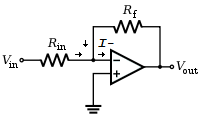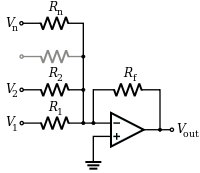# Inverting Opamp Amplifier

An inverting amplifier inverts and amplifies the input signal. As long as the op-amp gain is very large, the amplifier gain is determined by the external feedback resistor Rf and the input resistor Rin and not by op-amp parameters.If we apply KCL to the node at V- (the inverting input) and let the input current to the inverting input be I-, then \begin{equation} {{V_{out} - V_-} \over R_f } + {{V_{in} - V_-}\over R_{in} } = I_- \end{equation} Since the output is connected to V- through Rf, the opamp is in a negative feedback configuration. Thus \begin{equation} V_- = V_+ = 0 \end{equation} And assuming that I- is 0 and simplifying equation 1, \begin{equation} {{V_{out}} \over R_f } + {{V_{in}}\over R_{in} } = 0 \end{equation} Re-arrange the equation to obtain the gain of the inverting opamp amplifer \begin{equation} V_{out} = -{{R_f }\over {R_{in}}} {V_{in}} \end{equation}

# Summing Opamp Amplifier

The summing opamp amplifer is a general case of the inverting amplifier with multiple input voltages and resistors.Extending equation 3 to the multiple input voltages and resistors, \begin{equation} {{V_{out}} \over R_f } + {{V_{1}}\over R_{1} } + {{V_{2}}\over R_{2} } + ... + {{V_{n}}\over R_{n} } = 0 \end{equation} Re-arrange the equation to obtain the output voltage of the summing opamp amplifer \begin{equation} V_{out} = -R_f ( {{V_{1}}\over R_{1} } + {{V_{2}}\over R_{2} } + ... + {{V_{n}}\over R_{n} } ) \end{equation} In the case of R1 = R2 = .... = Rn = Rf, the output voltage is the sum of the input voltages \begin{equation} V_{out} = - ( V_{1} + V_{2} + ... + V_{n} ) \end{equation}

With advertising revenues falling despite increasing numbers of visitors, we need your help to maintain and improve this site, which takes time, money and hard work. Thanks to the generosity of our visitors who gave earlier, you are able to use this site at no charge.

If you have benefited from this site and are able, please give \$10 via Paypal. It will allow us continue into the future. It only takes a minute. Thanks!